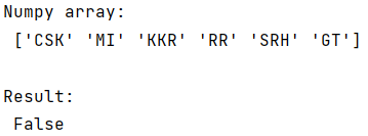# What is the most efficient way to check if a value exists in a NumPy array?

Given a NumPy array, we have to learn about the most efficient way to check if a value exists in it.
Submitted by Pranit Sharma, on June 23, 2022

NumPy is an abbreviated form of Numerical Python. It is used for different types of scientific operations in Python. Numpy is a vast library in python which is used for almost every kind of scientific or mathematical operation. It is itself an array that is a collection of various methods and functions for processing the arrays.

## Problem statement

Given a NumPy array, we have to learn about the most efficient way to check if a value exists in it.

## Most efficient way to check if a value exists in a NumPy array

To check if a value exists in a NumPy array or not, for this purpose, we will use any() method which will return True if the condition inside it is satisfied.

Note

To work with numpy, we need to import numpy package first, below is the syntax:

```import numpy as np
```

Let us understand with the help of an example,

## Python program to check if a value exists in a NumPy array

```# Importing numpy package
import numpy as np

# Creating a numpy array
arr = np.array(['CSK','MI','KKR','RR','SRH','GT'])

# Display array
print("Numpy array:\n",arr,"\n")

# Check if any value present in array
result = np.any(arr == 'RCB')

# Display result
print("Result:\n",result)
```

## Output

The output of the above program is: